Next: MINIMUM RECTANGLE TILING Up: Weighted Set Problems Previous: MINIMUM SUM OF SQUARES   Index

### MINIMUM ARRAY PARTITION

• INSTANCE: An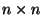array A of non-negative integers, and a positive integer p.
• SOLUTION: p-1 horizontal dividersand p-1 vertical dividers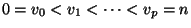partitioning A into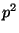blocks.
• MEASURE:• Good News: In APX .
• Bad News: Not approximable within less than 2 .
• Comment: The good news is valid also for generalizations to higher dimensions and other measures. The dual problem, where a limit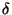is given instead of p, and where the problem is to find the minimum p such that the array can be partitioned intoblocks where each block's measure is bounded by, is approximable within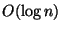.

Viggo Kann
2000-03-20